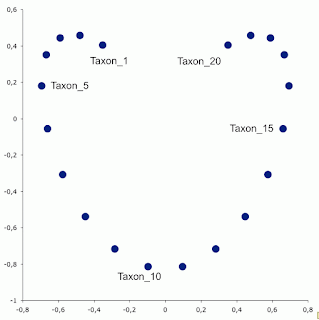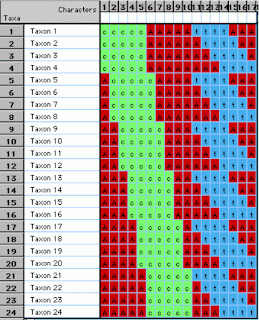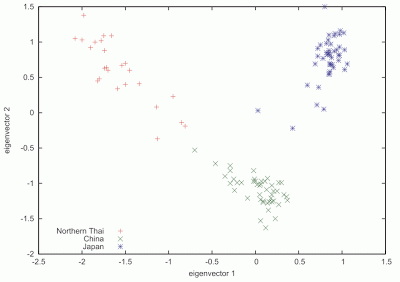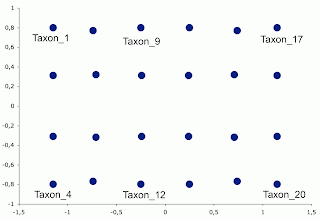## Wednesday, December 12, 2012

### Distortions and artifacts in Principal Components Analysis for analysis of genome data

It is perfectly clear from examples in the genomics literature that there is a problem occurring with the use of Principal Components Analysis (PCA) ordinations, and that it is apparently going undetected by users. This problem involves a spurious second axis in the output graph that is a curvilinear function of the first axis. The danger is that the ordination diagrams will be interpreted as showing biological patterns (see François et al. 2010; Arenas et al. 2013), when these patterns are not in the original datasets. I illustrate this problem here using some very simple datasets, and point to some examples of it in the literature. I then suggest some possible solutions, including the use of phylogenetic networks.

Introduction

Genomic data are multivariate, in the sense that we have multiple characters (the nucleotide sequences) for multiple organisms. "Ordination" is the term used when we arrange the multivariate data in a rational order, which is then usually displayed as a scatter plot, with the points representing the organisms. The spatial relationships of the points on the graph then indicate how similar the organisms are to each other (based on their genomes) — close points are more similar to each other than are distant points, as shown by the example in the first figure.Salmela et al. (2011), Figure 2d. Each dot represents the genome of one Swedish person, coloured according to their geographical location within northern Sweden.

There are many mathematical techniques for ordination, although they fall into two main groups: (i) eigenanalysis methods, and (ii) non-metric multidimensional scaling methods. These methods have a long history in some parts of biology, for example in ecology (Gauch 1982; Jongman et al. 1987; Kent & Coker 1992; Leps & Smilauer; Legendre & Legendre 2012), although they are virtually unknown in other parts. (Note: the eigenanalysis ordinations are sometimes confusingly called metric multidimensional scaling ordinations.)

The eigenanalysis method called Principal Components Analysis (PCA) was introduced by Patterson et al. (2006) to the study of genomic data, having previously been introduced into genetics by Menozzi et al. (1978)Cavalli-Sforza and Edwards (1964), and it has become quite popular in the literature since then. The basic idea is to summarize the data by calculating the correlation coefficient between each pair of organisms, and then to display as much as possible of the common pattern of variation along the first ordered axis of the graph, and as much of the remainder along the second axis, and so on. The different axes are expected to show independent patterns of genetic relationship among the organisms, with the first axis showing the most important pattern, the second axis the second-most important pattern, and so on.

However, ecologists have long known that the first two components of a PCA (and related eigenanalysis techniques such as correspondence analysis) often show an arching pattern, which is a mathematical artifact of the eigenanalysis rather than a real biological pattern in the original data (Gauch et al. 1977). This is most apparent when the sampling units cover a long ecological gradient and those samples at each end of the gradient have few species in common (Minchin 1987; see also this blog post). This is called the Arch Effect (or the Horseshoe Effect, or the Guttman Effect), in which the second axis is curved and twisted relative to the first axis, and thus the axes are not independent of each other.

The problem illustrated

Basically, the distortion involves the data pattern being displayed using one more axis than is needed for that data pattern. That is, a 1-dimensional pattern is displayed using 2 dimensions, instead, and a 2-dimensional pattern is displayed using 3 dimensions, etc. I will first illustrate this with a simple 1-dimensional pattern.

This first dataset consists of 20 nucleotide sequences. Each sequence consists of only two nucleotides (19 A and 5 C), and the pattern of Cs forms a simple gradient (ie. there is only one pattern in the data). This gradient can be displayed using only one ordination axis, which would arrange the taxa in order from Taxon_1 to Taxon_20. However, note that the patterns at the two ends of the gradient have nothing in common as far as the pattern of Cs is concerned.The gradient dataset.

The next figure is the Principal Components Analysis (PCA) ordination diagram of these data. Note that the 1-dimensional pattern is displayed using 2 dimensions, arranging the 20 taxa in an arch rather than in a straight line. (Note that the open part of the arch can face either up or down; in this case it happens to face up.) So, it appears in the scatter plot that Taxon_1 and Taxon_20 are rather similar to each other (ie. they are near each other), which is not true. Furthermore, the dots are not equally spaced, which they should be given the pattern in the original data. The second dimension, along with the unequal spacing of the points, is a mathematical artifact of the eignenanalysis rather than a pattern in the data. It is caused by the unimodal distribution of the pattern of Cs along the gradient.PCA ordination of the gradient data. Not all of the taxa are labelled.

The second dataset consists of 24 nucleotide sequences. Each sequence consists of three nucleotides (8 A, 5 C and 4 T), and the pattern of Cs forms one gradient while the pattern of Ts forms a second gradient (orthogonal to the first one). These two independent patterns can be displayed using two ordination axes, which would arrange the taxa in a 6x4 rectangular grid. (If this is not clear, look at the bottom two figures of this post.)The grid dataset.

This next figure is the PCA ordination diagram of these data. Note that the 2-dimensional pattern is displayed using 3 dimensions, only two of which are actually shown in the diagram. (It is standard to show only the first two axes, on the assumption that these contain most of the important information.) These axes arrange the 24 taxa in an arch in the first two dimensions, rather than in a straight line, and then separate pairs of taxa along the third axis. So, in two dimensions pairs of taxa appear to sit on top of one another, indicating a similarity that is not present in the original dataset. Once again, the use of the third dimension is an artifact of the eignenanalysis rather than a pattern in the data. It is caused by the unimodal distribution of the patterns of Cs and Ts along the two gradients.PCA ordination of the grid data.

Clearly, when the samples form gradients then eigenanalysis is not an appropriate ordination technique, although it is very useful under many other circumstances. This problem with gradients has previously been pointed out for genomic data when they form a clinal geographical pattern (Novembre & Stephens 2008; Reich et al. 2008), but clearly the problem involves much more than just geographical patterns. Also, the problem is being ignored by users — most of the examples below were published after the paper by Novembre & Stephens.

Literature examples

The Arch Effect has appeared repeatedly in the genomics literature (often produced using the SmartPCA, Plink or Eigenstrat computer programs), and some examples are illustrated here. Note that the axes are not always in the same orientation, but the arch should be found on axis 2 relative to axis 1, and it can be oriented either up or down.

These first two examples come from the very paper that first introduced PCA to genomics studies.Patterson et al. (2006), Figure 5.Patterson et al. (2006), Figure 8.

These next two examples are from studies of genomic data from Scandinavia.Salmela et al. (2011), Figure 2c.Skoglund et al. (2012), Figure 1c. Note that axis 1 is shown vertically.

The next two examples also come from worldwide genome studies. Note that the second of these refers to the ordination as metric multidimensional scaling (MDS).Stoneking & Krause (2011), Box 1a.Jakobsson et al. (2008), Figure 1d.

The next two examples also come from a worldwide genome study, but in this case the relationship of the Arch Effect to the geographical sampling is made explicit.Wang et al. (2012), Figure 1b.Wang et al. (2012), Figure 5b.

The next example illustrates rotation of the ordination axes, so that the arch is now aligned along the two axes.Novembre and Ramachandran (2011), Figure 4e.

Finally, the Gene Expression blog has an example, where the ordination is called a metric multidimensional scaling (MDS) ordination. This is based on ~3,000 individuals and 250,000 SNPs.

Possible solutions

There are three possible solutions to the Arch Effect problem in eigenanalysis ordinations.

First, one can try to mathematically correct the distortion. This approach was actively pursued in ecology via what is known as detrending and rescaling (Hill & Gauch 1980), in which the axes are forced to be independent of each other after the eigenanalysis. This approach works, in the sense that the Arch Effect disappears, but it has the drawback that all relationships between the axes are removed, including those that require more than one dimension to be displayed (Palmer 1993). So, while this method is still commonly encountered in the ecological literature, it is not uniformly recommended.

This approach is illustrated in the next figure, which is a Detrended Correspondence Analysis (DCA, with detrending by second-order polynomials) ordination analysis of the second of my simple datasets above. Note that the original 2-dimensional grid arrangement of the taxa is perfectly preserved, so that the ordination diagram is exactly as expected.DCA ordination of the grid data.

The second, and most obvious, solution to the problem is not to use an eigenanalysis ordination in the first place. That is, not all ordination techniques produce the same result. In this case we could use a Non-metric Multidimensional Scaling (NMDS) ordination, instead. The latter is a very different type of technique, mathematically, which explicitly tries to arrange the points in the ordination plot so that their relative distances reflect as closely as possible the pairwise "distances" in the original dataset (here, a distance is the inverse of a similarity). This technique is known to avoid the Arch Effect (Minchin 1987).

This is illustrated in the next figure, which is a NMDS ordination analysis of the second dataset. Note that the original 2-dimensional grid arrangement of the taxa is almost perfectly preserved. The main disadvantages of NMDS are the computational time required, the difficulty of finding the optimal solution for large datasets (eigenanalysis is significantly faster; Zheng et al. 2012), and the fact that the number of ordination axes needs to be specified beforehand.NMDS ordination of the grid data. Not all of the taxa are labelled.

The third solution to the problem is to use a phylogenetic network to display the multidimensional data, rather than using an ordination. Conceptually, this is similar to the NMDS type of ordination, but it uses a line graph to display the results rather than using a scatter plot. I have illustrated this in the next blog post (Networks can outperform PCA ordinations in phylogenetic analysis), where I report that this solution works quite well, too.

Conclusion

Every time you see an arch in a PCA plot, you might very well be looking at a mathematical artifact. You should bear this in mind when you interpret the ordination pattern, especially if you wish to draw biological conclusions from the diagram. You will, of course, see plenty of PCA ordinations without any evidence of an arch, and these are probably safe to interpret naively.

PCA can be useful when we expect genomes to be monotonically related to one another, but this is likely to be rather rare in nature. It is much more likely that genomes will have nonlinear relationships, such as unimodal patterns, with a peak at an intermediate part of any gradients that are present. Under these circumstances it produces artifacts, which may be mistakenly interpreted as representing real biological patterns. Under these circumstances, an alternative method of multivariate analysis should be used.

Note: updated Friday December 21 2012.

References

Arenas M, François O, Currat M, Ray N, Excoffier L (2013) Influence of admixture and paleolithic range contractions on current European diversity gradients. Molecular Biology and Evolution 30: 57-61.

Cavalli-Sforza L, Edwards AWF (1964) Analysis of human evolution. Proceedings of the 11th International Congress of Genetics, The Hague, 1963. Genetics Today 3: 923-933.

François O, Currat M, Ray N, Han E, Excoffier L, Novembre J (2010) Principal component analysis under population genetic models of range expansion and admixture. Molecular Biology and Evolution 27: 1257-1268.

Hill MO, Gauch HG (1980) Detrended Correspondence Analysis: an improved ordination technique. Vegetatio 42: 47-58.

Gauch HG (1982) Multivariate Analysis in Community Structure. Cambridge University Press, Cambridge.

Gauch HG, Whittaker RH, Wentworth TR (1977) A comparative study of reciprocal averaging and other ordination techniques. Journal of Ecology 65: 157-174.

Jongman RHG, ter Braak CJF, van Tongeren OFR (1987) Data Analysis in Community and Landscape Ecology. Pudoc, Wageningen.

Kent M, Coker P (1992) Vegetation Description and Analysis: a Practical Approach. Belhaven Press, London.

Legendre P, Legendre L (2012) Numerical Ecology, 3rd edition. Elsevier, Amsterdam.

Leps J, Smilauer P (2003) Multivariate Analysis of Ecological Data using Canoco. Cambridge University Press, Cambridge.

Menozzi P, Piazza A, Cavalli-Sforza L (1978) Synthetic maps of human gene frequencies in Europeans. Science 201: 786-792.

Minchin PR (1987) An evaluation of relative robustness of techniques for ecological ordinations. Vegetatio 69: 89-107.

Novembre J, Ramachandran S (2011) Perspectives on human population structure at the cusp of the sequencing era. Annual Review of Genomics and Human Genetics 12: 245-74.

Novembre J, Stephens M (2008) Interpreting principal component analyses of spatial population genetic variation. Nature Genetics 40: 646-649.

Palmer MW (1993) Putting things in even better order: the advantages of Canonical Correspondence Analysis. Ecology 74: 2215-2230.

Patterson N, Price AL, Reich D (2006) Population structure and eigenanalysis. PLoS Genetics 2: e190.

Reich D, Price AL, Patterson N (2008) Principal component analysis of genetic data. Nature Genetics 40: 491-492.

Salmela E, Lappalainen T, Liu J, Sistonen P, Andersen PM, Schreiber S, Savontaus ML, Czene K, Lahermo P, Hall P, Kere J (2011) Swedish population substructure revealed by genome-wide single nucleotide polymorphism data. PLoS One 6: e16747.

Skoglund P, Malmström H, Raghavan M, Storå J, Hall P, Willerslev E, Gilbert MT, Götherström A, Jakobsson M (2012) Origins and genetic legacy of Neolithic farmers and hunter-gatherers in Europe. Science 336: 466-469.

Stoneking M, Krause J (2011) Learning about human population history from ancient and modern genomes. Nature Reviews Genetics 12: 603-614.

Wang C, Zöllner S, Rosenberg NA (2012) A quantitative comparison of the similarity between genes and geography in worldwide human populations. PLoS Genetics 8: e1002886.

Zheng X, Levine D, Shen J, Gogarten SM, Laurie C, Weir BS (2012) A high-performance computing toolset for relatedness and Principal Component Analysis of SNP data. Bioinformatics 28: 3326-3328.